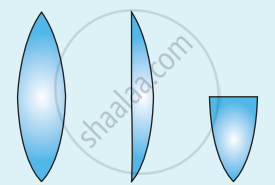Tamil Nadu Board of Secondary EducationHSC Science Class 12th

# A biconvex lens has focal length f and intensity of light I passing through it. What will be the focal length and intensity for portions of lenses obtained by cutting it vertically - Physics

Numerical

A biconvex lens has focal length f and intensity of light I passing through it. What will be the focal length and intensity for portions of lenses obtained by cutting it vertically and horizontally as shown in the figure?#### Solution

R1 = R

R2 = - R

Vertically-

1/"f" = (mu - 1)(1/("R"_1)_(-> "R") - 1/("R"_2)_(->-"R"))

1/"f" = (mu - 1) 2/"R"   ...(1)

1/"f"_"p" = (mu - 1)(1/(∞_(-> 0)) - (- 1/"R"))

1/"f"_"p" = (mu - 1)/"R"   ....(2)

equation (1) ÷ equation (2)

(1/"f")/(1/"f"_"p") = 2

"f"_"p"/"f" = 2

"f"_"p" = 2f

Horizontally-

1/"f'" = (mu - 1)(1/("R"_1)_(-> "R") - 1/("R"_2)_(->-"R"))

1/"f'" = (mu - 1) 2/"R"   ...(3)

f' = f

Concept: Spherical Mirrors
Is there an error in this question or solution?

Share